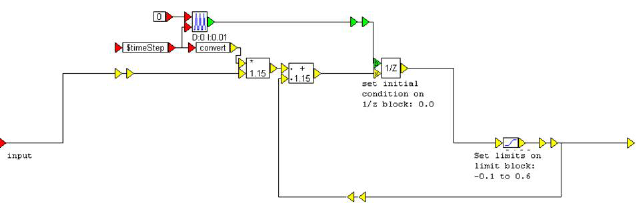## caseBlock Category: Nonlinear

Inputs:

case: The following rules apply to values fed into the case input:

The case input value must be scalar. If a non-integer value is fed into it, it is truncated. For example, 0.999 is truncated to 0.

If the case input value targets an out-of-range input, the case block returns an error. For example, an error results if the case input value is 5 for a four-element case block.

If the case input value targets an out-of-range input, but the default input has been enabled, then the output is the default input

If the case input value targets an unconnected input, the case block outputs 0.

x0 through xn: Inputs labeled 0 through n can be text strings, real or complex scalars, vectors, or matrices. They represent the possible execution paths. The maximum number of inputs is 125.

default: Lets you provide a value if the case index is outside the range of case input connectors. Input can be text strings, real or complex scalars, vectors, or matrices. For example, if you have case input connectors for 0 - 5, and index in with case value 6 or -27 then the output value of the case block will be the value input on the default connector. The default input is present only if Enable Default Case in the dialog is activated.

Description: The case block lets you specify an unlimited number of execution paths based on the case input value. The remaining inputs are the possible execution paths.

The main application of the case block is in the construction of large nested if-else decision structures, where regular if-else constructs using Boolean blocks become too cumbersome.

When you want to output a particular element in a matrix, use the index block. To perform time division, multiplex data streaming, use the demux block.Enable Default Case: Indicates the value if the case index is outside the range of case inputs.

#### Examples

1. Implementation of five scalar branches

Consider the decision tree:

If J = 0, then Y = A;

else

If J = 1, then Y = B;

else

If J = 2, then Y = C;

else

If J = 3, then Y = D;

else

If J = 4, then Y = E;

If A, B, C, D, and E are assumed to be constant values equal to 7, 14, 21, 28, and 35 respectively, the decision tree is realized as:Six const blocks produce values for the variable blocks named J, A, B, C, D, and E. The outputs of these variables are connected to a case block, with J connected to the case input, and variables A, B, C, D, and E connected to inputs 0, 1, 2, 3, and 4 respectively. The output of the case block is fed through a variable named Y and into a display block.

Since J is set to 3, the variable D is presented to the output as expected, and consequently, Y takes on the value of D, namely 28.

2. Implementation of three matrix branches

Consider the following part of the decision tree presented above:

If J = 0, then Y = A;

else

If J = 1, then Y = B;

else

If J = 2, then Y = C;

If you let A, B, and C be:the decision tree is realized as:Eight const blocks generate the elements of the three matrices, represented as three scalarToVec blocks. The variable J is set to 1 and is fed into the case input of a case block. The outputs of variables A, B, and C are wired to inputs 0, 1, and 2 of the case block. The output of the case block is connected to a variable Y, which is wired to a display block.

Since J is set to 1, the contents of variable B are presented at the output of the case block such that Y = B.

3. Implementation of three branches with a default valueIn this implementation, there are case input connectors for 0 - 2, and an index in with a unit ramp signal. When the value of the case input reaches 3, the output of the case block is the input on the default connector, which is 5.